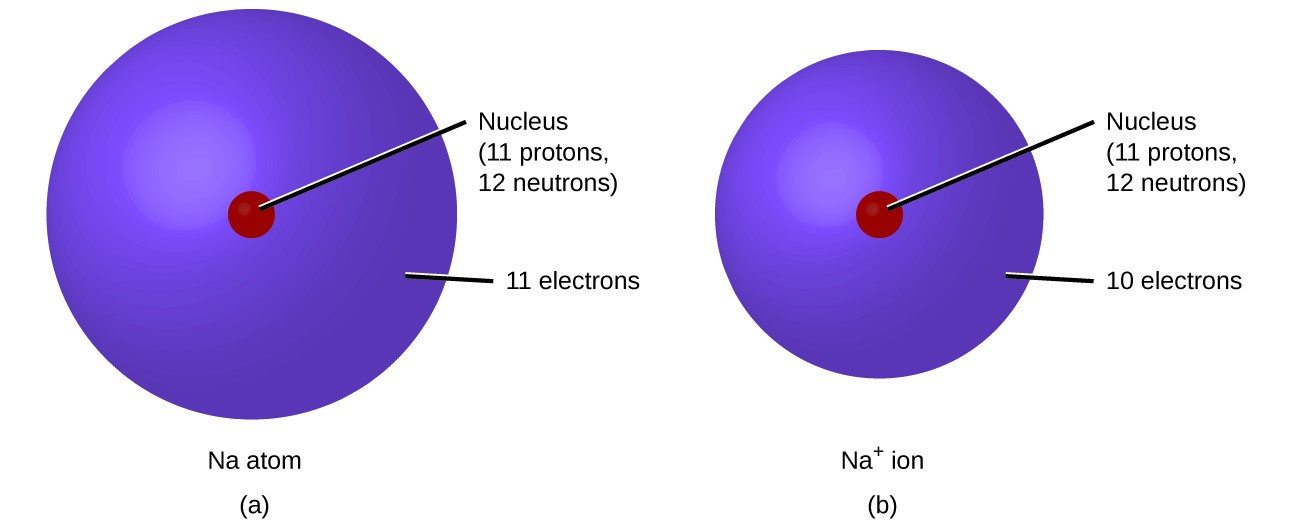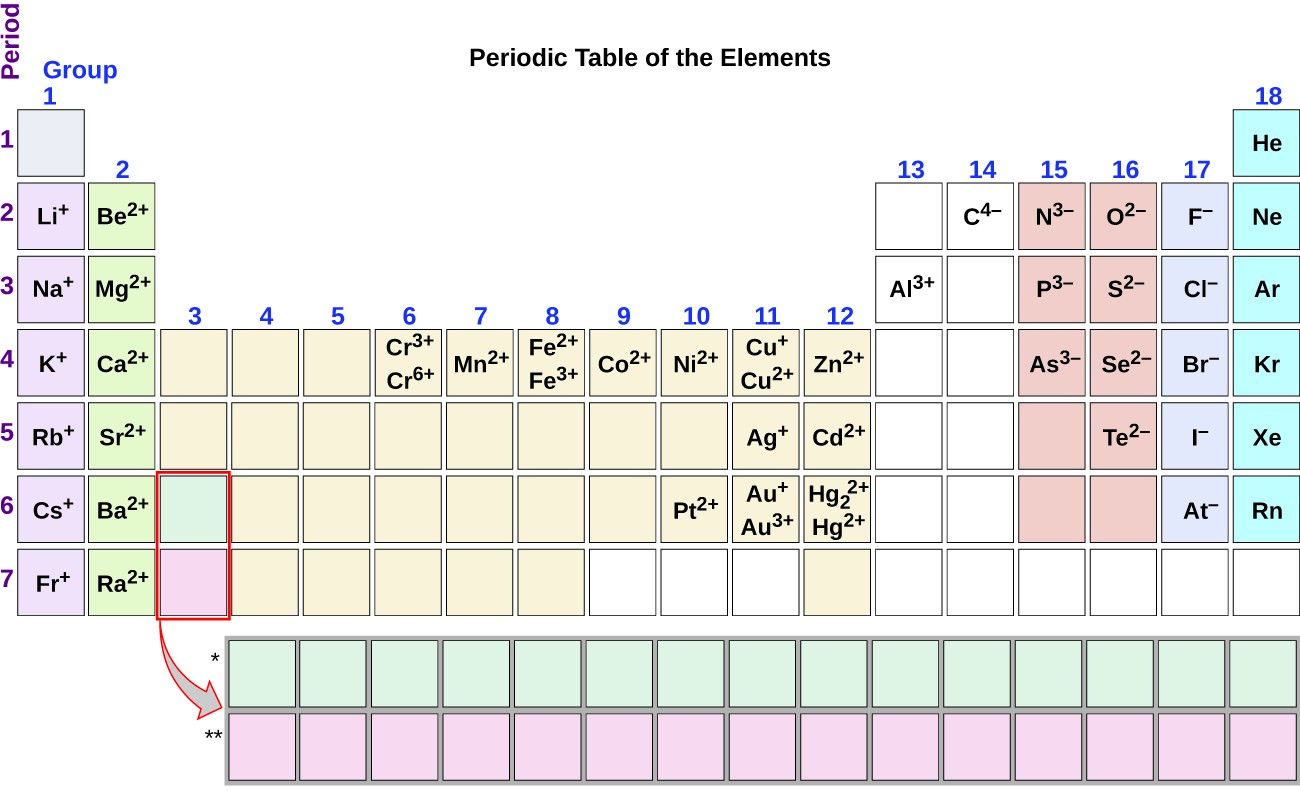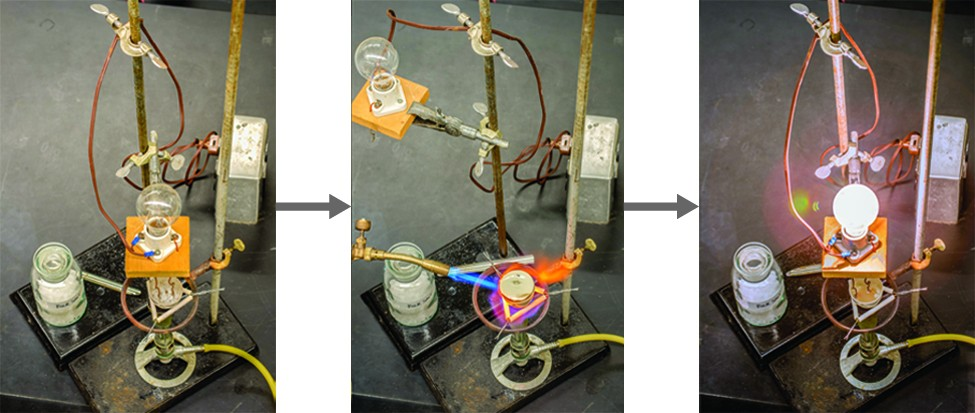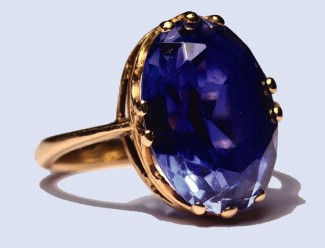## Molecular and Ionic Compounds

### Learning Outcomes

• Define ionic and molecular (covalent) compounds
• Predict the type of compound formed from elements based on their location within the periodic table
• Determine formulas for simple ionic compounds

In ordinary chemical reactions, the nucleus of each atom (and thus the identity of the element) remains unchanged. Electrons, however, can be added to atoms by transfer from other atoms, lost by transfer to other atoms, or shared with other atoms. The transfer and sharing of electrons among atoms govern the chemistry of the elements. During the formation of some compounds, atoms gain or lose electrons, and form electrically charged particles called ions (Figure 1).Figure 1. (a) A sodium atom (Na) has equal numbers of protons and electrons (11) and is uncharged. (b) A sodium cation (Na+) has lost an electron, so it has one more proton (11) than electrons (10), giving it an overall positive charge, signified by a superscripted plus sign.

You can use the periodic table to predict whether an atom will form an anion or a cation, and you can often predict the charge of the resulting ion. Atoms of many main-group metals lose enough electrons to leave them with the same number of electrons as an atom of the preceding noble gas. To illustrate, an atom of an alkali metal (group 1) loses one electron and forms a cation with a 1+ charge; an alkaline earth metal (group 2) loses two electrons and forms a cation with a 2+ charge, and so on. For example, a neutral calcium atom, with 20 protons and 20 electrons, readily loses two electrons. This results in a cation with 20 protons, 18 electrons, and a 2+ charge. It has the same number of electrons as atoms of the preceding noble gas, argon, and is symbolized $\text{Ca}^{2+}$. The name of a metal ion is the same as the name of the metal atom from which it forms, so $\text{Ca}^{2+}$ is called a calcium ion.

When atoms of nonmetal elements form ions, they generally gain enough electrons to give them the same number of electrons as an atom of the next noble gas in the periodic table. Atoms of group 17 gain one electron and form anions with a 1− charge; atoms of group 16 gain two electrons and form ions with a 2− charge, and so on. For example, the neutral bromine atom, with 35 protons and 35 electrons, can gain one electron to provide it with 36 electrons. This results in an anion with 35 protons, 36 electrons, and a 1− charge. It has the same number of electrons as atoms of the next noble gas, krypton, and is symbolized $\text{Br}^{-}$. (A discussion of the theory supporting the favored status of noble gas electron numbers reflected in these predictive rules for ion formation is provided in a later module of this text.)

Note the usefulness of the periodic table in predicting likely ion formation and charge (Figure 2). Moving from the far left to the right on the periodic table, main-group elements tend to form cations with a charge equal to the group number. That is, group 1 elements form 1+ ions; group 2 elements form 2+ ions, and so on. Moving from the far right to the left on the periodic table, elements often form anions with a negative charge equal to the number of groups moved left from the noble gases. For example, group 17 elements (one group left of the noble gases) form 1− ions; group 16 elements (two groups left) form 2− ions, and so on. This trend can be used as a guide in many cases, but its predictive value decreases when moving toward the center of the periodic table. In fact, transition metals and some other metals often exhibit variable charges that are not predictable by their location in the table. For example, copper can form ions with a 1+ or 2+ charge, and iron can form ions with a 2+ or 3+ charge.Figure 2. Some elements exhibit a regular pattern of ionic charge when they form ions.

### Example 1: Composition of Ions

An ion found in some compounds used as antiperspirants contains 13 protons and 10 electrons. What is its symbol?

Give the symbol and name for the ion with 34 protons and 36 electrons.

### Example 2: Formation of Ions

Magnesium and nitrogen react to form an ionic compound. Predict which forms an anion, which forms a cation, and the charges of each ion. Write the symbol for each ion and name them.

Aluminum and carbon react to form an ionic compound. Predict which forms an anion, which forms a cation, and the charges of each ion. Write the symbol for each ion and name them.

The ions that we have discussed so far are called monatomic ions, that is, they are ions formed from only one atom. We also find many polyatomic ions. These ions, which act as discrete units, are electrically charged molecules (a group of bonded atoms with an overall charge). Some of the more important polyatomic ions are listed in Table 1. Oxyanions are polyatomic ions that contain one or more oxygen atoms. At this point in your study of chemistry, you should memorize the names, formulas, and charges of the most common polyatomic ions. Because you will use them repeatedly, they will soon become familiar.

Table 1. Common Polyatomic Ions
Charge Name Formula Charge Name Formula
1+ ammonium ${\text{NH}}_{4}{}^{+}$ 1− permanganate ${\text{MnO}}_{4}{}^{-}$
1− acetate ${\text{C}}_{2}{\text{H}}_{3}{\text{O}}_{2}{}^{-}$ 1− hydrogen carbonate, or bicarbonate ${\text{HCO}}_{3}{}^{-}$
1− cyanide $\text{CN}^-$ 2− carbonate ${\text{CO}}_{3}{}^{2-}$
1− hydroxide $\text{OH}^-$ 2− peroxide ${\text{O}}_{2}{}^{2-}$
1− nitrate ${\text{NO}}_{3}{}^{-}$ 1− hydrogen sulfate, or bisulfate ${\text{HSO}}_{4}{}^{-}$
1− nitrite ${\text{NO}}_{2}{}^{-}$ 2− sulfate ${\text{SO}}_{4}{}^{2-}$
1− perchlorate ${\text{ClO}}_{4}{}^{-}$ 2− sulfite ${\text{SO}}_{3}{}^{2-}$
1− chlorate ${\text{ClO}}_{3}{}^{-}$ 1− dihydrogen phosphate ${\text{H}}_{2}{\text{PO}}_{4}{}^{-}$
1− chlorite ${\text{ClO}}_{2}{}^{-}$ 2− hydrogen phosphate ${\text{HPO}}_{4}{}^{2-}$
1− hypochlorite $\text{ClO}^-$ 3− phosphate ${\text{PO}}_{4}{}^{3-}$

Note that there is a system for naming some polyatomic ions; -ate and -ite are suffixes designating polyatomic ions containing more or fewer oxygen atoms. Per- (short for “hyper”) and hypo- (meaning “under”) are prefixes meaning more oxygen atoms than -ate and fewer oxygen atoms than -ite, respectively. For example, perchlorate is ${\text{ClO}}_{4}{}^{-}$, chlorate is ${\text{ClO}}_{3}{}^{-}$, chlorite is ${\text{ClO}}_{2}{}^{-}$ and hypochlorite is ${\text{ClO}^{-}}$. Unfortunately, the number of oxygen atoms corresponding to a given suffix or prefix is not consistent; for example, nitrate is ${\text{NO}}_{3}{}^{-}$ while sulfate is ${\text{SO}}_{4}{}^{2-}.$ This will be covered in more detail in the next module on nomenclature.

The nature of the attractive forces that hold atoms or ions together within a compound is the basis for classifying chemical bonding. When electrons are transferred and ions form, ionic bonds result. Ionic bonds are electrostatic forces of attraction, that is, the attractive forces experienced between objects of opposite electrical charge (in this case, cations and anions). When electrons are “shared” and molecules form, covalent bonds result. Covalent bonds are the attractive forces between the positively charged nuclei of the bonded atoms and one or more pairs of electrons that are located between the atoms. Compounds are classified as ionic or molecular (covalent) on the basis of the bonds present in them.

## Ionic Compounds

When an element composed of atoms that readily lose electrons (a metal) reacts with an element composed of atoms that readily gain electrons (a nonmetal), a transfer of electrons usually occurs, producing ions. The compound formed by this transfer is stabilized by the electrostatic attractions (ionic bonds) between the ions of opposite charge present in the compound. For example, when each sodium atom in a sample of sodium metal (group 1) gives up one electron to form a sodium cation, Na+, and each chlorine atom in a sample of chlorine gas (group 17) accepts one electron to form a chloride anion, Cl, the resulting compound, NaCl, is composed of sodium ions and chloride ions in the ratio of one Na+ ion for each Cl ion. Similarly, each calcium atom (group 2) can give up two electrons and transfer one to each of two chlorine atoms to form CaCl2, which is composed of Ca2+ and Cl ions in the ratio of one Ca2+ ion to two Cl ions.

A compound that contains ions and is held together by ionic bonds is called an ionic compound. The periodic table can help us recognize many of the compounds that are ionic: When a metal is combined with one or more nonmetals, the compound is usually ionic. This guideline works well for predicting ionic compound formation for most of the compounds typically encountered in an introductory chemistry course. However, it is not always true (for example, aluminum chloride, AlCl3, is not ionic).

You can often recognize ionic compounds because of their properties. Ionic compounds are solids that typically melt at high temperatures and boil at even higher temperatures. For example, sodium chloride melts at 801 °C and boils at 1413 °C. (As a comparison, the molecular compound water melts at 0 °C and boils at 100 °C.) In solid form, an ionic compound is not electrically conductive because its ions are unable to flow (“electricity” is the flow of charged particles). When molten, however, it can conduct electricity because its ions are able to move freely through the liquid (Figure 3).Figure 3. Sodium chloride melts at 801 °C and conducts electricity when molten. (credit: modification of work by Mark Blaser and Matt Evans)

Watch this video to see a mixture of salts melt and conduct electricity. (Note that the video has no narration. You can access the audio description using the widget below the video.)

In every ionic compound, the total number of positive charges of the cations equals the total number of negative charges of the anions. Thus, ionic compounds are electrically neutral overall, even though they contain positive and negative ions. We can use this observation to help us write the formula of an ionic compound. The formula of an ionic compound must have a ratio of ions such that the numbers of positive and negative charges are equal.

### Example 3: Predicting the Formula of an Ionic Compound

The gemstone sapphire (Figure 4) is mostly a compound of aluminum and oxygen that contains aluminum cations, Al3+, and oxygen anions, O2−. What is the formula of this compound?Figure 4. Although pure aluminum oxide is colorless, trace amounts of iron and titanium give blue sapphire its characteristic color. (credit: modification of work by Stanislav Doronenko)

Predict the formula of the ionic compound formed between the sodium cation, Na+, and the sulfide anion, S2−.

Many ionic compounds contain polyatomic ions (Table 1) as the cation, the anion, or both. As with simple ionic compounds, these compounds must also be electrically neutral, so their formulas can be predicted by treating the polyatomic ions as discrete units. We use parentheses in a formula to indicate a group of atoms that behave as a unit. For example, the formula for calcium phosphate, one of the minerals in our bones, is $\text{Ca}_{3}(\text{PO}_{4})_{2}$. This formula indicates that there are three calcium ions $(\text{Ca}^{2+})$ for every two phosphate $\left({\text{PO}}_{4}{}^{3-}\right)$ groups. The ${\text{PO}}_{4}{}^{3-}$ groups are discrete units, each consisting of one phosphorus atom and four oxygen atoms, and having an overall charge of 3-. The compound is electrically neutral, and its formula shows a total count of three Ca, two P, and eight O atoms.

### Example 4: Predicting the Formula of a Compound with a Polyatomic Anion

Baking powder contains calcium dihydrogen phosphate, an ionic compound composed of the ions Ca2+ and ${\text{H}}_{2}{\text{PO}}_{4}{}^{-}$. What is the formula of this compound?

Predict the formula of the ionic compound formed between the lithium ion and the peroxide ion, ${\text{O}}_{2}{}^{2-}$ (Hint: Use the periodic table to predict the sign and the charge on the lithium ion.)

Because an ionic compound is not made up of single, discrete molecules, it may not be properly symbolized using a molecular formula. Instead, ionic compounds must be symbolized by a formula indicating the relative numbers of its constituent cations. For compounds containing only monatomic ions (such as $\text{NaCl}$) and for many compounds containing polyatomic ions (such as $\text{CaSO}_{4})$, these formulas are just the empirical formulas introduced earlier. However, the formulas for some ionic compounds containing polyatomic ions are not empirical formulas. For example, the ionic compound sodium oxalate is comprised of $\text{Na}^{+}$ and ${\text{C}}_{2}{\text{O}}_{4}{}^{2-}$ ions combined in a 2:1 ratio, and its formula is written as $\text{Na}_{2}\text{C}_{2}\text{O}_{4}$. The subscripts in this formula are not the smallest-possible whole numbers, as each can be divided by 2 to yield the empirical formula, $\text{NaCO}_{2}$. This is not the accepted formula for sodium oxalate, however, as it does not accurately represent the compound’s polyatomic anion, ${\text{C}}_{2}{\text{O}}_{4}{}^{2-}$.

### Ionic Bonding

Ionic bonds form between metals and nonmetals.

You can view the transcript for “Ionic Bonding Part 2” here (opens in new window).

## Molecular Compounds

Many compounds do not contain ions but instead consist solely of discrete, neutral molecules. These molecular compounds (covalent compounds) result when atoms share, rather than transfer (gain or lose), electrons. Covalent bonding is an important and extensive concept in chemistry, and it will be treated in considerable detail in a later module of this text. We can often identify molecular compounds on the basis of their physical properties. Under normal conditions, molecular compounds often exist as gases, low-boiling liquids, and low-melting solids, although many important exceptions exist.

Whereas ionic compounds are usually formed when a metal and a nonmetal combine, covalent compounds are usually formed by a combination of nonmetals. Thus, the periodic table can help us recognize many of the compounds that are covalent. While we can use the positions of a compound’s elements in the periodic table to predict whether it is ionic or covalent at this point in our study of chemistry, you should be aware that this is a very simplistic approach that does not account for a number of interesting exceptions. Shades of gray exist between ionic and molecular compounds, and you’ll learn more about those later.

### Example 5: Predicting the Type of Bonding in Compounds

Predict whether the following compounds are ionic or molecular:

1. KI, the compound used as a source of iodine in table salt
2. H2O2, the bleach and disinfectant hydrogen peroxide
3. CHCl3, the anesthetic chloroform
4. Li2CO3, a source of lithium in antidepressants

Using the periodic table, predict whether the following compounds are ionic or covalent:

1. SO2
2. CaF2
3. N2H4
4. Al2(SO4)3

### Key Concepts and Summary

Metals (particularly those in groups 1 and 2) tend to lose the number of electrons that would leave them with the same number of electrons as in the preceding noble gas in the periodic table. By this means, a positively charged ion is formed. Similarly, nonmetals (especially those in groups 16 and 17, and, to a lesser extent, those in Group 15) can gain the number of electrons needed to provide atoms with the same number of electrons as in the next noble gas in the periodic table. Thus, nonmetals tend to form negative ions. Positively charged ions are called cations, and negatively charge ions are called anions. Ions can be either monatomic (containing only one atom) or polyatomic (containing more than one atom).

Compounds that contain ions are called ionic compounds. Ionic compounds generally form from metals and nonmetals. Compounds that do not contain ions, but instead consist of atoms bonded tightly together in molecules (uncharged groups of atoms that behave as a single unit), are called covalent compounds. Covalent compounds usually form from two nonmetals.

### Try It

1. Using the periodic table, predict whether the following chlorides are ionic or covalent: KCl, NCl3, ICl, MgCl2, PCl5, and CCl4.
2. Using the periodic table, predict whether the following chlorides are ionic or covalent: SiCl4, PCl3, CaCl2, CsCl, CuCl2, and CrCl3.
3. For each of the following compounds, state whether it is ionic or covalent. If it is ionic, write the symbols for the ions involved:
1. NF3
2. BaO,
3. (NH4)2CO
4. Sr(H2PO4)2
5. IBr
6. Na2O
4. For each of the following compounds, state whether it is ionic or covalent, and if it is ionic, write the symbols for the ions involved:
1. KClO4
2. MgC2H3O2
3. H2S
4. Ag2S
5. N2Cl4
6. Co(NO3)2
5. For each of the following pairs of ions, write the symbol for the formula of the compound they will form:
1. Ca2+, S2-
2. ${\text{NH}}_{4}{}^{+}$, ${\text{SO}}_{4}{}^{2-}$
3. Al3+, Br–  (d) Na+, ${\text{HPO}}_{4}{}^{2-}$  (e) Mg2+, ${\text{PO}}_{4}{}^{3-}$
6. For each of the following pairs of ions, write the symbol for the formula of the compound they will form:
1. K+, O2-
2. ${\text{NH}}_{4}{}^{+}$, ${\text{PO}}_{4}{}^{3-}$
3. Al3+, O2-
4. Na+, ${\text{CO}}_{3}{}^{2-}$
5. Ba2+, ${\text{PO}}_{4}{}^{3-}$

## Glossary

covalent bond: attractive force between the nuclei of a molecule’s atoms and pairs of electrons between the atoms

covalent compound: (also, molecular compound) composed of molecules formed by atoms of two or more different elements

ionic bond: electrostatic forces of attraction between the oppositely charged ions of an ionic compound

ionic compound: compound composed of cations and anions combined in ratios, yielding an electrically neutral substance

molecular compound: (also, covalent compound) composed of molecules formed by atoms of two or more different elements

monatomic ion: ion composed of a single atom

oxyanion: polyatomic anion composed of a central atom bonded to oxygen atoms

polyatomic ion: ion composed of more than one atom